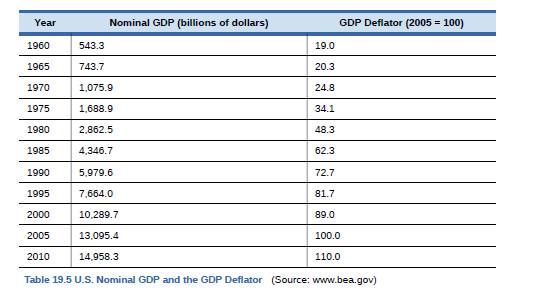Chapter 19, Problem 3SCQ### Principles of Economics 2e

2nd Edition
Steven A. Greenlaw; David Shapiro
ISBN: 9781947172364

#### Solutions

Chapter
Section### Principles of Economics 2e

2nd Edition
Steven A. Greenlaw; David Shapiro
ISBN: 9781947172364
Textbook Problem

# Using data from Table 19.5 how much of the nominal GDP growth from 1980 to 1990 was real GDP and how much was inflation?To determine

To ascertain the part of GDP rise from 1980 to 1990 that is due to rise in real GDP and the part of rise in GDP from 1980 to 1990 that is due to inflation.

Explanation

We are given the GDP for two periods as follows:

GDP (1980) = $2,862.5 billion GDP (1990) =$5,979.6 billion

Using the formula for GDP, we calculate the real GDP for the two periods as follows:

a)RealGDPin1980GDPDeflator=NominalGDP RealGDP/100RealGDP=NominalGDPGDPDeflator×100RealGDP=2,862.548.3×100RealGDP(1980)=$5926.5billion b)RealGDPin1990GDPDeflator=NominalGDP RealGDP/100RealGDP=NominalGDPGDPDeflator×100RealGDP=5,979.672.7×100RealGDP(1980)=$8,225billion

Thus, the change in nominal and real GDP is calculated as follows.

ChangeinNominalGDPbetween1980and1990=NominalGDP(1990)NominalGDP(1980)=5,979

### Still sussing out bartleby?

Check out a sample textbook solution.

See a sample solution

#### The Solution to Your Study Problems

Bartleby provides explanations to thousands of textbook problems written by our experts, many with advanced degrees!

Get Started